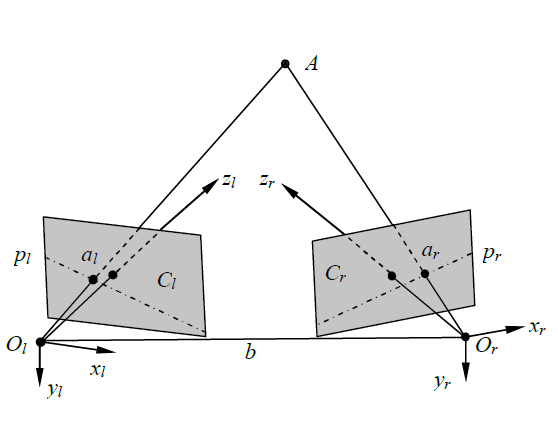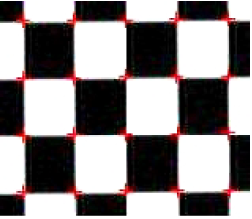@Pigmon 2017-04-05T11:14:06.000000Z 字数 5668 阅读 148

# The Comparison of Calibration Method of Binocular Stereo Vision System

文献阅读

3rd International Conference on Material, Mechanical and Manufacturing Engineering (IC3ME 2015) © 2015. The authors - Published by Atlantis Press

Ke Zhang *, Zhao Gao

# 双目立体视觉系统中摄像机标定方法的比较

## 建立双目视觉系统## 基于遗传算法进行标定

$X_w$方向（mm） $Y_w$方向（mm） $Z_w$方向（mm）

## 基于BP神经网络进行标定BP神经网络的训练结果如表2所示。

$\Delta X$ $\Delta Y$ $\Delta Z$ $\Delta X$ $\Delta Y$ $\Delta Z$
0.0548 0.0529 0.2744 0.2533 0.2467 0.9561

$\Delta X$ $\Delta Y$ $\Delta Z$ $\Delta X$ $\Delta Y$ $\Delta Z$
0.0579 0.0536 0.2972 0.3197 0.2739 1.3578

## 参考文献

$$ Marr D.Vision.W.H.Freeman and Company, San Francisco. Chinese version: computational theory of vision. G.Z. Yao，L.Liu and Y.J.Wang，Science Press. Beijing, China, 1988, pp. 6-37(In Chinese).
$$ S.Y. You and G.Y. Xu: Journal of Image and Graphics. Vol. 2, No. 1, (1997), pp. 17~24(In Chinese).
$$ Y.D. Jia: Machine Vision. Science Press. Beijing, China, 2000, pp. 1-25(In Chinese).
$$ S.D. Ma and Z.Y. Zhang: Computer Vision-Fundamentals of Computational Theory and Algorithms. Science Press. Beijing, China, 1998, pp. 72-93(In Chinese).
$$ M. Kong and S.M. Wang: Journal of Metrology. Vol. 25, No. 4, (2004), pp. 294~297(In Chinese).
$$ M. Zhou and S.D Sun: Principle and Application of Genetic Algorithm. National Defense Industry Press. Beijing, China, 2002, pp. 4-64(In Chinese).
$$ J. Qiang and Y.M. Zhang: IEEE Transactions on Systems, Man, and Cybernetics－Part A: Systems and Humans. Vol. 31, No. 2, (2001), pp. 120~130.
$$ Roberts M and Naftel A J: Genetic Algorithms in Image Processing and Vision. IEE Colloquium on, 1994: 12/1~12/5
$$ G.Q. Wei and S.D. Ma: IEEE Transactions on Pattern Analysis and Machine Intelligence. Vol. 16, No. 5, (1994), pp. 469~480.• 私有
• 公开
• 删除